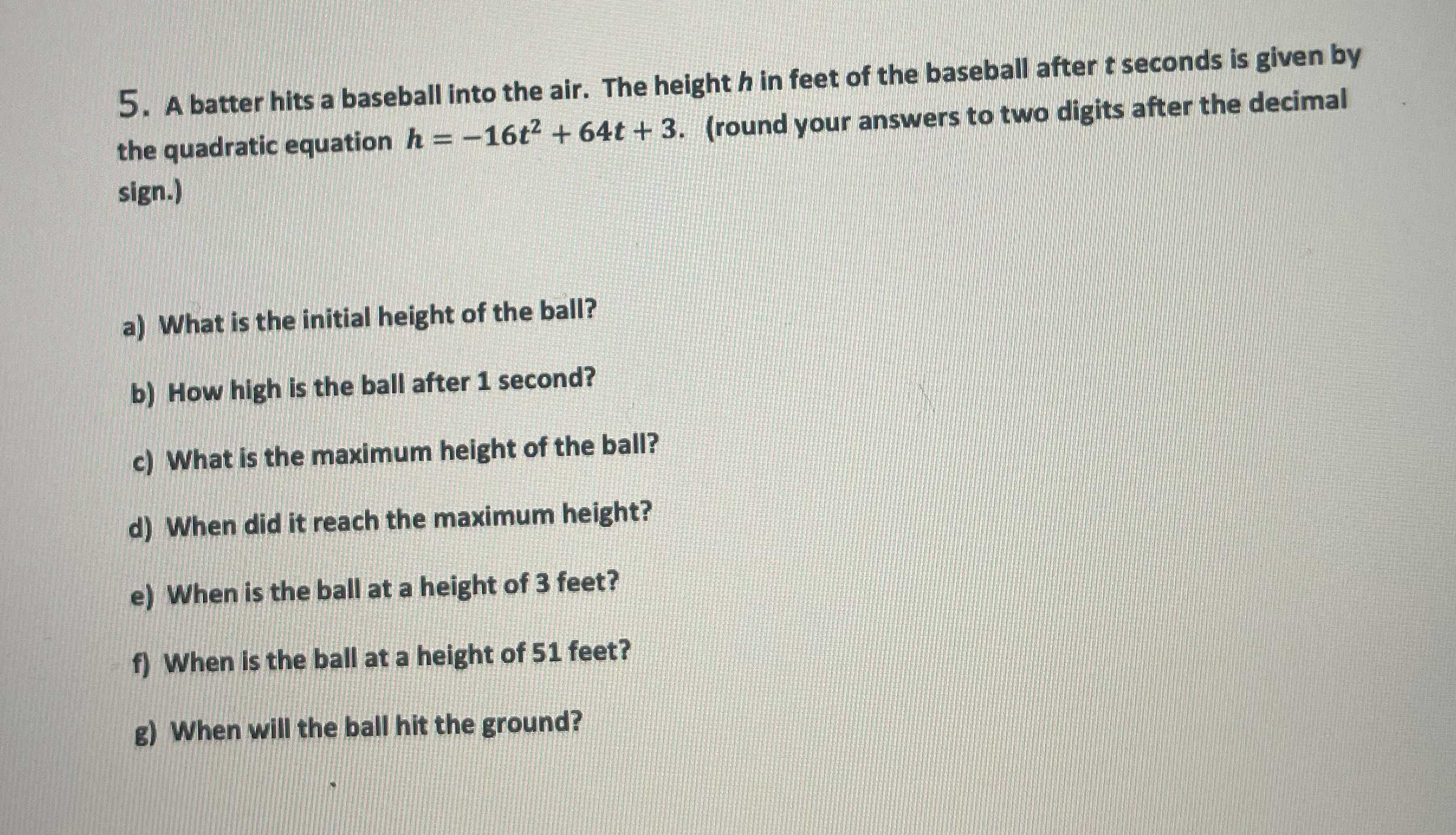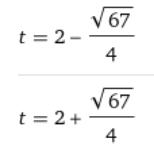### ¿Todavía tienes preguntas de matemáticas?

Pregunte a nuestros tutores expertos
Algebra
PreguntaA batter hits a baseball into the air. The height $$h$$ in feet of the baseball after $$t$$ seconus give the quadratic equation $$h = - 16 t ^ { 2 } + 64 t + 3$$ . (round your answers to two digits after the decimal sign.)

a) What is the initial height of the ball?

b) How high is the ball after $$1$$ second?

c) What is the maximum height of the ball?

d) When did it reach the maximum height?

e) When is the ball at a height of $$3$$ feet?

f) When is the ball at a height of $$51$$ feet?

g) When will the ball hit the ground?

a)t=0, h=3

b)t=1, h=51

c)h=67

d)2

e)t=0 or t=2

f)t=1 or t=3

g)Solución
View full explanation on CameraMath App.